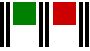Trading Indicators - what are they?A trading indicator is any variable that gives information useful to a trader. Indicators are the most commonly encountered thing in commodity trading, although they are not always referred to as indicators.

Indicators are used almost exclusively as part of the rules for entering and exiting markets.

There are very many indicators, here are some common ones:

Average True Range

How much does a market move, say in a day?

It had a low of 200 the day before yesterday and a high of 205, so it moved 5 points. Yesterday it had a low of 208 and a high of 210, therefore it move 2 points. 5 points plus 2 points is 7 points so it seems to be an average of 3½ points over the two days.

But you saw what happened – the market ‘gapped’ from its close on day 1 because its low price on day 2 was three points above the previous day’s close.
With a high of 210 on the second day, a span of 10 points has been covered over the two days, averaging 5 points per day.

All this sort of tedious stuff has to be considered when you want to find out how the market prices have been ranging.

Because prices do not usually open on the following day at the point where they closed on the previous day, it was necessary to solve such problems by inventing the true range indicator!

The true range indicator takes account of market ‘gapping’ and gives a true reading of the amount a market ‘moves’ in a day.

The true range is invariably used as an average true range indicator by calculating the average over a time period of a number of days (time intervals).

Moving Average

The moving average is one of the most popular indicators used as it is simple to calculate, informative and very versatile.

Moving averages of the closing price are generally used by commodity traders but any others can be used.

By calculating the moving average over different numbers of days (time intervals) and comparing this with the moving average for other time periods, an impression of ‘what the price has been doing’ can be formed. The shorter the time interval, the more reactive to change the MA will be and so this gives rise to other indicators that compare two MAs of different invervals.

What are known as moving average crossover systems have been developed that buy when a shorter interval MA crosses a longer interval one and sell when the opposite occurs.

Such systems can be very effective when they are used in conjunction with sound methods and money management techniques.

Mid Point Price

The calculation of the mid point price is simply the average of the high and low price for the time interval concerned.

A mid point price line could be used in calculations in place of say, a closing price line.

Price Oscillator

This indicator employs the common technique of subtracting a longer term moving average from a shorter term one. When the difference is positive i.e. the shorter term average is greater, it can be regarded as a ‘buy’ signal: when the result is negative, a ‘sell’ can be interpreted.

Bollinger Bands

The Upper and Lower Bollinger bands form a ‘channel’ in which each band is an equal but varying distance from a moving average price line. The amount by which the band is distant from this ‘middle’ line is directly related to the standard deviation of the price for that day.

Because the standard deviation varies every day, so does the distance where the upper and lower bands are set. The bands expand and contract according to how the standard deviation fluctuates and as the SD is a measure of volatility, the bands widen when the market is more volatile and narrow when it is less volatile.

Trading systems typically buy the market when the price breaks out above the upper band and sell when it breaks down below the lower band. Of course, the trader can modify the actual rules by adding filters and also varying the number of standard deviations used to calculate the band distance.

As with moving average indicators, time intervals of any length can be chosen to apply to Bollinger Bands, for example, if a 25 day period is selected, then the typical method would be to calculate a 25 day moving average of the closing price for the middle band and then calculate the standard deviation of the closing price over the 25 day period.

This is the normal SD calculation. Any number of SDs can be chosen as the offsetting distance. If, say 2 SDs is chosen, then for the upper band this value in points is added to the middle band value. For the lower band, the identical amount is subtracted.

Calculation of indicators

Indicators are nearly always calculated directly or indirectly from market prices. Some indicators are very simple, such as the Middle Price, whereas others e.g. Bollinger Bands are more complicated.

What is important is that you understand the concept of the indicator and although it is perfectly OK for you to ask how it is calculated, the detailed number crunching is best left to your computer.Contact DavidDavid Bromley helps
new and aspiring systems